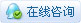72809浏览

# [教程] Mind+Python编程入门系列课程 — 09 弹球小游戏积分版Mind+Python编程入门系列课程第九课——弹球小游戏积分版

Python编程入门系列课程——01 智能绘星
Python编程入门系列课程——02 模拟星空
Python编程入门系列课程——03 龟龟赛跑
Python编程入门系列课程——04小海龟走迷宫
Python编程入门系列课程——05飞机大战小游戏
Python编程入门系列课程——06飞机大战彩蛋版
Python编程入门系列课程——07 桌面小挂件
Python编程入门系列课程 — 08 弹球小游戏

经过上节课的学习，我们了解了python中的逻辑运算符、学习了列表的索引以及类的实例方法的创建。同时，也学会了使用tkinter库实现键盘交互的方法。在上节课中，我们利用tkinter库及python知识设计了一个弹球小游戏，那么这节课，我们将在上节课的基础上，进一步优化弹球小游戏，使其更加有趣起来!

1、掌握python中常见的赋值运算符

1、掌握使用tkinter库在界面上实时计算得分的方法

1、电脑
2、mind+编程软件

1.添加积分功能及启动和停止按键
2.设置游戏在不同时间段的难度不同

1、任务分析2、程序编写

STEP1：创建与保存项目文件

STEP2：创建与保存python文件

STEP3：编写程序
``````import tkinter  #引入GUI工具包turtle库
import random   #引入随机函数
import time     #引入时间库

#创建一个界面和配置界面的一些基本属性
window = tkinter.Tk()#创建一个tk的窗口实例对象
window.title('Game')#设置窗口标题为game
window.resizable(0, 0)#通知窗口管理器调整布局大小,0,0表示不能被拉伸
window.wm_attributes('-topmost', 1)#窗口永远在前
#创建一个500x400的界面,背景色为浅蓝色，边框为厚度为0
canvas = tkinter.Canvas(window, width=500, height=400, highlightthickness=0,bg='light green')
canvas.pack()#安置画布

#初始界面，画布上绘制文字
startsigal=canvas.create_text(430,10,text='DOWN:START',font=('Times',10),state='normal')
endsigal=canvas.create_text(430,30,text='UP:END',font=('Times',10),state='normal')
leftsigal=canvas.create_text(430,50,text='LEFT:LEFT',font=('Times',10),state='normal')
rightsigal=canvas.create_text(430,70,text='RIGHT:RIGHT',font=('Times',10),state='normal')

over=canvas.create_text(250,200,text='GAME OVER',font=('Times',30),state='hidden')
start=canvas.create_text(430,110,text='GAME START',font=('Times',10),state='normal')
score=canvas.create_text(430,90,text='SCORE:',font=('Times',10),state='hidden')

def __init__(self,canvas,color):#创建init方法
self.canvas=canvas #初始化canvas画布
#保存tkinter画球拍时返回的id，为后期移动屏幕上的球拍做准备，create_rectangle 画矩形，fill为球拍的颜色
self.id=canvas.create_rectangle(0,0,150,10,fill=color)#画一个矩形并记录下它的id
self.canvas.move(self.id,150,380)#将球拍移动至初始位置
self.started=False#球拍是否开始移动，True表示开始运行
self.x=0#设置对象变量x，初始值为0.也就是球拍先不移动
self.canvas_width=self.canvas.winfo_width()#球拍的画布宽度铺满
self.canvas.bind_all("<KeyPress-Left>",self.turn_left)
self.canvas.bind_all("<KeyPress-Right>",self.turn_right)
self.canvas.bind_all("<KeyPress-Down>", self.game_start)#向下键开始
self.canvas.bind_all("<KeyPress-Up>", self.game_stop)#向上键停止

#创建实例方法，是指在类中定义的函数。
def turn_left(self,evt):#左移，evt是向系统注册的事件
self.x=-4#左移速度为4
def turn_right(self,evt):#右移
self.x=4#右移速度为4
def game_start(self,evt):#开始
self.started=True
def game_stop(self,evt):#停止
self.started = False

def draw(self):#创建draw()方法
self.canvas.move(self.id,self.x,0)#纵坐标固定，横坐标可移动
self.x=0
self.x=0

class Ball():#创建球类
'''创建Ball类，初始化对象，即创建对象设置属性,一个canvas也就是画图用来画一个球，一个是color，表示球的颜色
init函数是在对象被创建的同时就设置属性的一种方法，Python会在创建新对象时自动调用这个函数。'''
self.canvas=canvas#初始化canvas
'''保存tkinter画小球返回的id，为后期移动屏幕上的小球做准备，参数分别表示为:(10,10)
表示左上角x,y坐标，(25,25)表示右下角x,y坐标，即创建一个直径为15的圆,fill为小球的填充色'''
self.id=canvas.create_oval(10,10,25,25,fill=color)#画圆并记录下它的id
self.canvas.move(self.id,245,100)#将小球移动到初始位置，初始位置可通过传参进行更改，有默认值
starts=[-3,-2,-1,1,2,3]# 给一串x分量的起始值（x和y代表横坐标和纵坐标的分量）
random.shuffle(starts)# shuffle()方法将序列的所有元素随机排序
self.x=starts# 随机混排序，赋值给对象变量x，让它起始的时候获得随机分量值，横向速度不同，引起球每次起始角度都不同
self.y=-7 #向上移动
self.hit_bottom = False# 小球是否碰触到画布底部，初始值为False，即没有碰到

def draw(self):#让球出现在主界面上
self.canvas.move(self.id, self.x, self.y)# 让小球可以水平和垂直运动
pos = self.canvas.coords(self.id)# coords函数通过id返回画布球的坐标列表
# 判断小球是否撞到画布顶部或者底部，保证小球反弹回去，不消失
if pos>= 400:#如果小球的右下角y坐标大于画布宽度，则表示小球碰到了画布底部，游戏结束
self.hit_bottom=True
if pos >= 500:#如果小球的右下角x坐标大于等于画布宽度，则向左移速为5
self.x=-5
if pos<=0:#如果小球的左上角x坐标小于等于0，则向右移速为5
self.x=5
if pos<=0:#如果小球的左上角y坐标小于0，则向下移速为5
self.y=5

if self.hit_paddle(pos)==True:# 判断 球 是否碰到了 球拍，如果碰到了则使小球回弹
self.y=-5#向上移动三像素

#该函数用于判断 球 是否碰到了 球拍，如果碰到了则使小球回弹，否则游戏结束
# 如果小球的右下角x坐标 大于等于 球拍左上角x坐标，且小球左上角x坐标 小于等于 球拍右下角x坐标
# 如果小球右下角y坐标 大于等于 球拍左上角y坐标，且小球右下角y坐标 小于等于 球拍右下角y坐标
return True#返回值为真
else:
return False#返回值为假

#得分计算
class Score:#创建得分类

def __init__(self,canvas,color):
self.score=0#初始化得分为0
self.canvas=canvas# 把参数canvas赋值给对象变量canvas
canvas.itemconfig(score, state='normal')#修改组件属性，将得分展示在文本控件中
self.id=canvas.create_text(470,90,text=self.score,fill=color)#创建文本控件，用户保存得分

self.score+=1#自增一分
self.canvas.itemconfig(self.id,text=self.score)

score=Score(canvas,'black')#创建得分实例
while True:

if  ball.hit_bottom==False:#游戏开始且小球未触底
ball.draw()#调用球的draw函数
else:
canvas.itemconfig(start, state='hidden')#修改组件属性
canvas.itemconfig(over, state='normal')#修改组件属性

window.update()#更新屏幕
time.sleep(0.01)#程序推迟执行0.01秒

3、程序运行运算符 说明 举例 展开形式 += 加赋值 x+=y x=x+y

self.score+=1#自增一分

 运算符 说明 举例 展开形式 = 简单的赋值运算 x=y x=y += 加赋值 x+=y x=x+y -= 减赋值 x-=y x=x-y *= 乘赋值 x*=y x=x*y /= 除赋值 x/=y x=x/y %= 取余数赋值 x%=y x=x%y **= 幂赋值 x**=y x=x**y //= 最整除赋值 x//=y x=x//y
 乐一乐    至此，弹球小游戏积分第一版我们就完成啦，赶紧来试一试吧，看看你最高能得多少分呢?

1、任务分析2、程序编写
STEP1：创建与保存python文件

STEP2：编写程序

``````import tkinter#导入tkinter库
#创建一个界面和配置界面的一些基本属性
import tkinter#引入GUI工具包turtle库
import random#引入随机函数
import time#引入时间库

global speed#创建全局变量speed

#创建一个界面和配置界面的一些基本属性
window = tkinter.Tk()#创建一个tk的窗口实例对象
window.title('Game')#设置窗口标题为game
window.resizable(0, 0)#通知窗口管理器调整布局大小,0,0表示不能被拉伸
window.wm_attributes('-topmost', 1)#窗口永远在前
#创建一个500x400的界面,背景色为浅蓝色，边框为厚度为0
canvas = tkinter.Canvas(window, width=500, height=400, highlightthickness=0,bg='light green')
canvas.pack()#安置画布

#初始界面，画布上绘制文字
startsigal=canvas.create_text(430,10,text='DOWN:START',font=('Times',10),state='normal')
endsigal=canvas.create_text(430,30,text='UP:END',font=('Times',10),state='normal')
leftsigal=canvas.create_text(430,50,text='LEFT:LEFT',font=('Times',10),state='normal')
rightsigal=canvas.create_text(430,70,text='RIGHT:RIGHT',font=('Times',10),state='normal')

over=canvas.create_text(250,200,text='GAME OVER',font=('Times',30),state='hidden')
start=canvas.create_text(430,110,text='GAME START',font=('Times',10),state='normal')
score=canvas.create_text(430,90,text='SCORE:',font=('Times',10),state='hidden')
victory=canvas.create_text(250,200,text='Victory',font=('Times',30),state='hidden')

def __init__(self,canvas,color):#创建init方法
self.canvas=canvas #初始化canvas画布
# 保存tkinter画球拍时返回的id，为后期移动屏幕上的球拍做准备，create_rectangle 画矩形，fill为球拍的颜色
self.id=canvas.create_rectangle(0,0,150,10,fill=color)#画一个矩形并记录下它的id
self.canvas.move(self.id,150,380)# 将球拍移动至初始位置
self.started=False#球拍是否开始移动，True表示开始运行
self.x=0# 设置对象变量x，初始值为0.也就是球拍先不移动
self.canvas_width=self.canvas.winfo_width()#球拍的画布宽度铺满
self.canvas.bind_all("<KeyPress-Left>",self.turn_left)
self.canvas.bind_all("<KeyPress-Right>",self.turn_right)
self.canvas.bind_all("<KeyPress-Down>", self.game_start)#向下键开始
self.canvas.bind_all("<KeyPress-Up>", self.game_stop)#向上键停止

#创建实例方法，是指在类中定义的函数。
def turn_left(self,evt):#左移，evt是向系统注册的事件
self.x=-4#左移速度为4
def turn_right(self,evt):#右移
self.x=4#右移速度为4
def game_start(self,evt):#开始
self.started=True
def game_stop(self,evt):#停止
self.started = False

def draw(self):#创建draw()方法
self.canvas.move(self.id,self.x,0)#纵坐标固定，横坐标可移动
self.x=0
self.x=0

class Ball():#创建球类
'''创建Ball类，初始化对象，即创建对象设置属性,一个canvas也就是画图用来画一个球，一个是color，表示球的颜色
init函数是在对象被创建的同时就设置属性的一种方法，Python会在创建新对象时自动调用这个函数。'''
self.canvas=canvas#初始化canvas
'''保存tkinter画小球返回的id，为后期移动屏幕上的小球做准备，参数分别表示为:(10,10)
表示左上角x,y坐标，(25,25)表示右下角x,y坐标，即创建一个直径为15的圆,fill为小球的填充色'''
self.id=canvas.create_oval(10,10,25,25,fill=color)#画圆并记录下它的id
self.canvas.move(self.id,245,100)# 将小球移动到初始位置，初始位置可通过传参进行更改，有默认值
starts=[-3,-2,-1,1,2,3]# 给一串x分量的起始值（x和y代表横坐标和纵坐标的分量）
random.shuffle(starts)# shuffle()方法将序列的所有元素随机排序
self.x=starts# 随机混排序，赋值给对象变量x，让它起始的时候获得随机分量值，横向速度不同，引起球每次起始角度都不同
self.y=-3 #向上移动
self.hit_bottom = False# 小球是否碰触到画布底部，初始值为False，即没有碰到

def draw(self):#让球出现在主界面上
self.canvas.move(self.id, self.x, self.y)# 让小球可以水平和垂直运动
pos = self.canvas.coords(self.id)# coords函数通过id返回画布球的坐标列表

if score.score >= 0and score.score<=2:#得分在0-2分内
speed = 3#设置移速为3
elif score.score >= 3and score.score<=5:#得分在3-5分内
speed  = 4
elif score.score >= 6and score.score<=8:#得分在6-8分内
speed  = 5
elif score.score >= 9and score.score<=15:#得分在9-15分内
speed  = 6
else :#其他情况
speed  = 7

# 判断小球是否撞到画布顶部或者底部，保证小球反弹回去，不消失
if pos>= 400:# 如果小球的右下角y坐标大于画布宽度，则表示小球碰到了画布底部，游戏结束
self.hit_bottom=True
if pos >= 500:#如果小球的右下角x坐标 大于等于画布宽度，则向左移速为
self.x=-speed
if pos<=0:#如果小球的左上角x坐标 小于等于0，则向右移速为
self.x=speed
if pos<=0:#如果小球的左上角y坐标小于0，则向下移速为
self.y=speed

if self.hit_paddle(pos)==True:# 判断 球 是否碰到了 球拍，如果碰到了则使小球回弹
self.y=-speed #向上移动三像素

#该函数用于判断 球 是否碰到了 球拍，如果碰到了则使小球回弹，否则游戏结束
# 如果小球的右下角x坐标 大于等于 球拍左上角x坐标，且小球左上角x坐标 小于等于 球拍右下角x坐标
# 如果小球右下角y坐标 大于等于 球拍左上角y坐标，且小球右下角y坐标 小于等于 球拍右下角y坐标
return True#返回值为真
else:
return False#返回值为假

#得分计算
class Score:#创建得分类

def __init__(self,canvas,color):
self.score=0#初始化得分为0
self.canvas=canvas# 把参数canvas赋值给对象变量canvas
canvas.itemconfig(score, state='normal')# 修改组件属性，将得分展示在文本控件中
self.id=canvas.create_text(470,90,text=self.score,fill=color)#创建文本控件，用户保存得分

self.score+=1#自增一分
self.canvas.itemconfig(self.id,text=self.score)
if self.score == 18:
canvas.itemconfig(victory, state='normal')#修改组件属性
canvas.itemconfig(over, state='hidden')#修改组件属性

score=Score(canvas,'black')#创建得分实例
while True:

if  ball.hit_bottom==False:#游戏开始且小球未触底
ball.draw()#调用球的draw函数
else:
canvas.itemconfig(start, state='hidden')#修改组件属性
canvas.itemconfig(over, state='normal')#修改组件属性
window.update()#强制更新屏幕
time.sleep(0.01)#程序推迟执行0.01秒

3、程序运行乐一乐    至此，弹球小游戏积分第二版我们就完成啦，你需要多少次才能获胜呢? 之后，尝试改变移速进一步增加难度再试试吧！

 程序 Python知识点 添加积分件程序设计 常见的赋值运算符 变化移速程序设计 无

想一想，除了加快小球的移动速度，我们还能如何控制游戏的难度呢？！（可参考方向：缩短球拍的长度，改变移速，球拍位置等）

 您需要登录后才可以回帖 登录 | 立即注册 本版积分规则 回帖并转播 回帖后跳转到最后一页

[[wsData.name]]

#### 硬件清单

• [[d.name]]